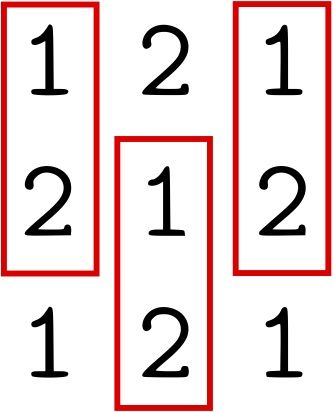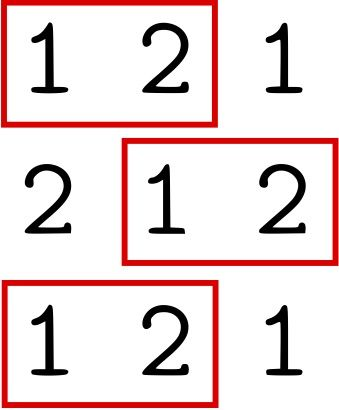시간 제한 메모리 제한 제출 정답 맞은 사람 정답 비율
1 초 512 MB 8 7 7 87.500%

## 문제

Given a permutation of numbers from 1 to n, find a square matrix conforming to the following rules:

1. The matrix should include only numbers from the permutation;
2. The given permutation should occur in every row of the matrix as a contiguous subsequence, read from left to right;
3. The given permutation should occur in every column of the matrix as a contiguous subsequence, read from top to bottom;
4. The matrix size is the smallest possible.

## 입력

The first line of input is a positive integer n ≤ 500.

The second line of input consists of n space-separated integers: the permutation itself.

## 출력

The first line of output should be an integer m: the size of the matrix. The next m lines should list m consecutive rows of the matrix. Each of these lines should contain m integers separated by spaces: the values in the corresponding row.

The size m should be minimum possible. If there are several possible answers, print any one of them.

## 예제 입력 1

2
1 2


## 예제 출력 1

3
1 2 1
2 1 2
1 2 1


## 힌트

Here is where the permutation occurs in the matrix from the example: